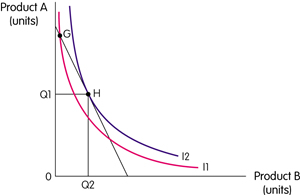#cfa #cfa-level-1 #economics #has-images #microeconomics #reading-14-demand-and-supply-analysis-consumer-demand #subject-4-consumer-equilibrium-maximizing-utility-subject-to-the-budget-contraint
The budget constraint line separates consumption bundles that are attainable from those that are unattainable. A consumer will maximise utility by consuming on the highest possible indifference curve (i.e., we assume all income is spent). This is where an indifference curve is tangent to the highest possible budget line.A consumer could consume at G, for example, but would be on a higher indifference curve at H. This means that to maximise utility the consumer would consume Q1 of product A and Q2 of product B.

The consumer is maximising utility where the budget line and indifference curve are tangent, i.e., MUB/MUA = PB/PA.

If you want to change selection, open document below and click on "Move attachment"

Subject 4. Consumer Equilibrium: Maximizing Utility Subject to the Budget Constraint
The budget constraint line separates consumption bundles that are attainable from those that are unattainable. A consumer will maximise utility by consuming on the highest possible indifference curve (i.e., we assume all income is spent). This is where an indifference curve is tangent to the highest possible budget line. A consumer could consume at G, for example, but would be on a higher indifference curve at H. This means that to maximise utility the consumer would consume Q1 of product A and Q2 of product B. The consumer is maximising utility where the budget line and indifference curve are tangent, i.e., MU B /MU A = P B /P A . An Increase in Income An increase in income shifts the budget line out parallel. The new combinations of products that maximise utility can be id